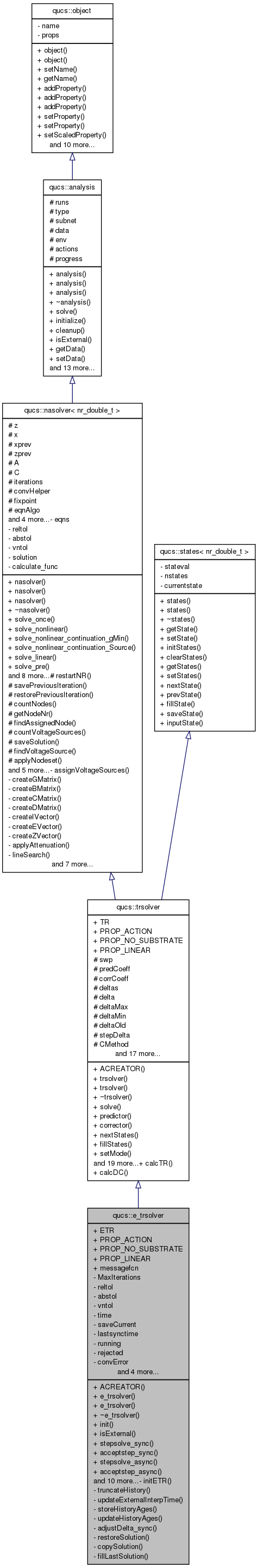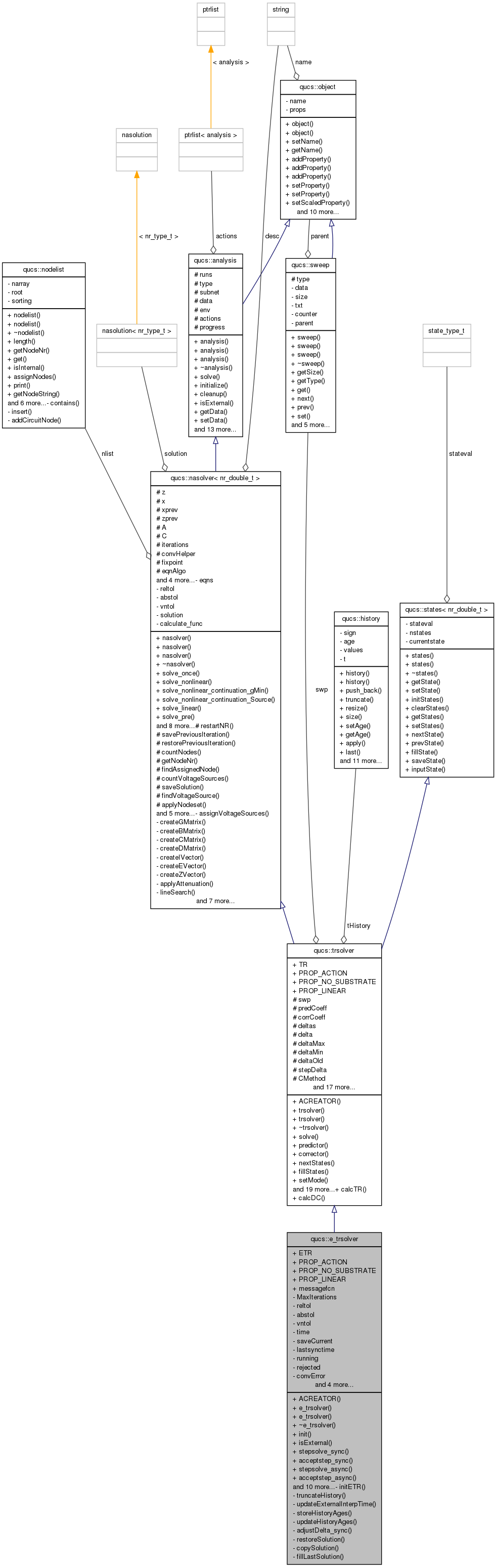Qucs-core  0.0.19
qucs::e_trsolver Class Reference

External interface class for transient simulation. More...

`#include <e_trsolver.h>`

Inheritance diagram for qucs::e_trsolver:[legend]
Collaboration diagram for qucs::e_trsolver:[legend]

Public Member Functions

ACREATOR (e_trsolver)
e_trsolver (char *)
e_trsolver (e_trsolver &)
~e_trsolver ()
int init (nr_double_t, nr_double_t, int)
bool isExternal ()
informs whether this is an external sim
int stepsolve_sync (nr_double_t synctime)
void acceptstep_sync (void)
int stepsolve_async (nr_double_t steptime)
void acceptstep_async (void)
void rejectstep_async (void)
void getsolution (double *)
int setECVSVoltage (char *ecvsname, nr_double_t V)
Sets the voltage of an exterally controlled voltage source.
int getJacRows ()
Returns the number of rows in the Jacobian matrix for the circuit.
int getJacCols ()
Returns the number of columns in the Jacobian matrix for the circuit.
void getJacData (int r, int c, nr_double_t &data)
Obtains the data from the Jacobian matrix for the circuit.
int getNodeV (char *label, nr_double_t &nodeV)
Obtains the voltage of a node by name.
int getVProbeV (char *probename, nr_double_t &probeV)
Obtains the voltage reported by a voltage probe.
int getIProbeI (char *probename, nr_double_t &probeI)
Obtains the current reported by a current probe.
void debug (void)
void printx (void)

Data Fields

ETR
PROP_ACTION
PROP_NO_SUBSTRATE
PROP_LINEAR
void(* messagefcn )(int level, const char *format,...)
Pointer to function used to print messages during a sim.

Private Member Functions

void initETR (nr_double_t start, nr_double_t, int)
void truncateHistory (nr_double_t)
void updateExternalInterpTime (nr_double_t)
void storeHistoryAges (void)
void updateHistoryAges (nr_double_t)
void restoreSolution (void)
void copySolution (tvector< nr_double_t > *, tvector< nr_double_t > *)
void fillLastSolution (tvector< nr_double_t > *)

Private Attributes

int MaxIterations
nr_double_t reltol
nr_double_t abstol
nr_double_t vntol
nr_double_t time
nr_double_t saveCurrent
nr_double_t lastsynctime
int running
int rejected
int convError
std::vector< nr_double_tinitialhistages
tvector< nr_double_t > * lastsolution 
nr_double_t lastasynctime
nr_double_t lastdeltas 
nr_double_t lastdelta

Detailed Description

External interface class for transient simulation.

This class provides access to the transient solving functionality of Qucs for external software.

Definition at line 1104 of file e_trsolver.cpp.

Constructor & Destructor Documentation

 qucs::e_trsolver::e_trsolver ( char * n )

Definition at line 107 of file e_trsolver.cpp.

 qucs::e_trsolver::e_trsolver ( e_trsolver & o )

Definition at line 151 of file e_trsolver.cpp.

 qucs::e_trsolver::~e_trsolver ( )

Definition at line 124 of file e_trsolver.cpp.

Member Function Documentation

 void qucs::e_trsolver::acceptstep_async ( void )

Definition at line 780 of file e_trsolver.cpp.

 void qucs::e_trsolver::acceptstep_sync ( void )

Definition at line 507 of file e_trsolver.cpp.

 qucs::e_trsolver::ACREATOR ( e_trsolver )
 void qucs::e_trsolver::adjustDelta_sync ( nr_double_t t ) ` [private]`

Definition at line 555 of file e_trsolver.cpp.

 void qucs::e_trsolver::copySolution ( tvector< nr_double_t > * src, tvector< nr_double_t > * dest ) ` [private]`

Definition at line 826 of file e_trsolver.cpp.

 void qucs::e_trsolver::debug ( void )

Definition at line 161 of file e_trsolver.cpp.

 void qucs::e_trsolver::fillLastSolution ( tvector< nr_double_t > * s ) ` [private]`

Definition at line 285 of file e_trsolver.cpp.

 int qucs::e_trsolver::getIProbeI ( char * probename, nr_double_t & probeI )

Obtains the current reported by a current probe.

Parameters:
 probename Pointer to character array containing the name of the probe probeI Reference to nr_double_t in which the current will be returned
Returns:
Integer flag reporting success or failure

This method searches for the current probe with the given name in probename. If the probe exists, the value of the probe current is copied to probeI. Returns 0 if the probe with the given name was found and -1 otherwise.

Definition at line 949 of file e_trsolver.cpp.

 int qucs::e_trsolver::getJacCols ( )

Returns the number of columns in the Jacobian matrix for the circuit.

Definition at line 1066 of file e_trsolver.cpp.

 void qucs::e_trsolver::getJacData ( int r, int c, nr_double_t & data )

Obtains the data from the Jacobian matrix for the circuit.

Parameters:
 r Pointer to character array containing the name of the voltage c Reference to nr_double_t in which the node voltage will be returned
Returns:
The Jacobian matrix data at the specified location.

Definition at line 1071 of file e_trsolver.cpp.

 int qucs::e_trsolver::getJacRows ( )

Returns the number of rows in the Jacobian matrix for the circuit.

Definition at line 1061 of file e_trsolver.cpp.

 int qucs::e_trsolver::getNodeV ( char * label, nr_double_t & nodeV )

Obtains the voltage of a node by name.

Parameters:
 label Pointer to character array containing the name of the voltage nodeV Reference to nr_double_t in which the node voltage will be returned
Returns:
Integer flag reporting success or failure

This method searches for the node with the given name in label. If the node exists, the value of the voltage at that node is copied to nodeV. Returns 0 if the node was found, and -1 otherwise.

Definition at line 887 of file e_trsolver.cpp.

 void qucs::e_trsolver::getsolution ( double * lastsol )

Definition at line 873 of file e_trsolver.cpp.

 int qucs::e_trsolver::getVProbeV ( char * probename, nr_double_t & probeV )

Obtains the voltage reported by a voltage probe.

Parameters:
 probename Pointer to character array containing the name of the probe probeV Reference to nr_double_t in which the node voltage will be returned
Returns:
Integer flag reporting success or failure

This method searches for the voltage probe with the given name in probename. If the probe exists, the value of the probe voltage is copied to probeV. Returns 0 if the probe with the given name was found and -1 otherwise.

Definition at line 903 of file e_trsolver.cpp.

 int qucs::e_trsolver::init ( nr_double_t start, nr_double_t firstdelta, int mode )

Definition at line 180 of file e_trsolver.cpp.

 void qucs::e_trsolver::initETR ( nr_double_t start, nr_double_t firstdelta, int mode ) ` [private]`

Definition at line 292 of file e_trsolver.cpp.

 bool qucs::e_trsolver::isExternal ( void ) ` [inline, virtual]`

informs whether this is an external sim

External simulations will be ignored by qucsator. This function is used to determine whether the analysis is external or not.

Reimplemented from qucs::analysis.

Definition at line 66 of file e_trsolver.h.

 void qucs::e_trsolver::printx ( void )

Definition at line 370 of file e_trsolver.cpp.

 void qucs::e_trsolver::rejectstep_async ( void )

Definition at line 798 of file e_trsolver.cpp.

 void qucs::e_trsolver::restoreSolution ( void ) ` [private]`
 int qucs::e_trsolver::setECVSVoltage ( char * ecvsname, nr_double_t V )

Sets the voltage of an exterally controlled voltage source.

Parameters:
 ecvsname The name of the voltage source to be set. V New value of the voltage.
Returns:
Integer flag reporting success or failure.

This method searches for the ecvs component with the given name in ecvsname. If the ecvs exists, the value of the voltage to be set at the next time step is set to the supplied value in V.

You can set the voltage of ecvs components in subcircuits created by using a name up of the subcircuit heirarchy it is in, e.g. the name

SUBtop.SUBlower.ECVS1

to set the voltage of an ecvs named ECVS1 which is located in subcircuit SUBlower, which is itself in subcircuit SUBtop in the top level circuit.

Returns 0 if the ecvs was found, and -1 otherwise.

Definition at line 990 of file e_trsolver.cpp.

 int qucs::e_trsolver::stepsolve_async ( nr_double_t steptime )

Definition at line 637 of file e_trsolver.cpp.

 int qucs::e_trsolver::stepsolve_sync ( nr_double_t synctime )

Definition at line 407 of file e_trsolver.cpp.

 void qucs::e_trsolver::storeHistoryAges ( void ) ` [private]`

Definition at line 272 of file e_trsolver.cpp.

 void qucs::e_trsolver::truncateHistory ( nr_double_t t ) ` [private]`

Definition at line 1051 of file e_trsolver.cpp.

 void qucs::e_trsolver::updateExternalInterpTime ( nr_double_t t ) ` [private]`

Definition at line 1031 of file e_trsolver.cpp.

 void qucs::e_trsolver::updateHistoryAges ( nr_double_t newage ) ` [private]`

Definition at line 840 of file e_trsolver.cpp.

Field Documentation

 nr_double_t qucs::e_trsolver::abstol` [private]`

Reimplemented from qucs::nasolver< nr_type_t >.

Definition at line 175 of file e_trsolver.h.

 int qucs::e_trsolver::convError` [private]`

Definition at line 182 of file e_trsolver.h.

 qucs::e_trsolver::ETR

Definition at line 1105 of file e_trsolver.cpp.

 std::vector qucs::e_trsolver::initialhistages` [private]`

Definition at line 197 of file e_trsolver.h.

 nr_double_t qucs::e_trsolver::lastasynctime` [private]`

Definition at line 199 of file e_trsolver.h.

 nr_double_t qucs::e_trsolver::lastdelta` [private]`

Definition at line 201 of file e_trsolver.h.

 nr_double_t qucs::e_trsolver::lastdeltas` [private]`

Definition at line 200 of file e_trsolver.h.

 tvector* qucs::e_trsolver::lastsolution` [private]`

Definition at line 198 of file e_trsolver.h.

 nr_double_t qucs::e_trsolver::lastsynctime` [private]`

Definition at line 179 of file e_trsolver.h.

 int qucs::e_trsolver::MaxIterations` [private]`

Definition at line 173 of file e_trsolver.h.

 void(* qucs::e_trsolver::messagefcn)(int level, const char *format,...)

Pointer to function used to print messages during a sim.

Parameters:
 level Inter flag indicating the log level format Formatting string in the same format as printf ... Additional arguments to the printing function

By default this points to the standard Qucs logprint function (found in logging.c) on creation of an e_trsolver instance. The log level flag indicates whether the message is a status update or an error message. format contains the formatting string in the same style as printf (when using logprint). The additional arguments are used by logprint in the supplied formatting string.

Definition at line 169 of file e_trsolver.h.

Reimplemented from qucs::trsolver.

Definition at line 1105 of file e_trsolver.cpp.

Reimplemented from qucs::trsolver.

Definition at line 1105 of file e_trsolver.cpp.

Reimplemented from qucs::trsolver.

Definition at line 1105 of file e_trsolver.cpp.

 int qucs::e_trsolver::rejected` [private]`

Reimplemented from qucs::trsolver.

Definition at line 181 of file e_trsolver.h.

 nr_double_t qucs::e_trsolver::reltol` [private]`

Reimplemented from qucs::nasolver< nr_type_t >.

Definition at line 174 of file e_trsolver.h.

 int qucs::e_trsolver::running` [private]`

Definition at line 180 of file e_trsolver.h.

 nr_double_t qucs::e_trsolver::saveCurrent` [private]`

Definition at line 178 of file e_trsolver.h.

 nr_double_t qucs::e_trsolver::time` [private]`

Definition at line 177 of file e_trsolver.h.

 nr_double_t qucs::e_trsolver::vntol` [private]`

Reimplemented from qucs::nasolver< nr_type_t >.

Definition at line 176 of file e_trsolver.h.

The documentation for this class was generated from the following files: